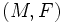# Flag curvature

## Definition

The flag curvature of a Finsler manifold$(M,F)$ (viz a differential manifold$M$ endowed with a Finsler metric$F$) defines a scalar (real number) for the following input: a point on the manifold, a tangent plane at that point, and a nonzero vector in the tangent plane.

Fill this in later

When the Finsler metricis a Riemannian metric, the flag curvature depends only on the tangent plane and not on the nonzero vector. Further, it equals the sectional curvature of that tangent plane.